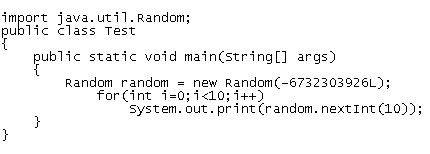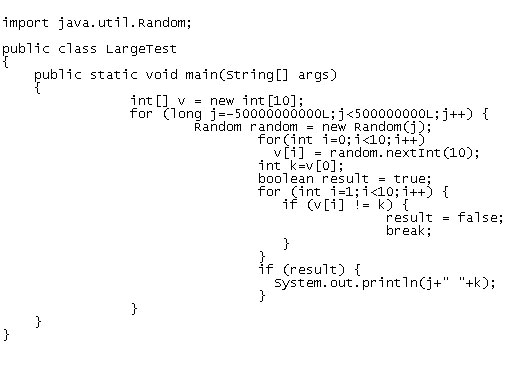All programming languages have random number generator classes or libraries, which produce sequences of random numbers. Those sequences are similar to pi in that they run on forever (well, sort of). As Harold explains to a class of bored teenagers in this Person of Interest clip, because pi runs forever (though so far only 10 trillion digits have been computed) every number or word that exists can be found within it. The same can be said of sequences of random numbers. And since Java's random number generator is algorithmic, to get it to return a specific number we need only to find it in the sequence. Let's take a look at how this works.Many pseudo random number generators (PRNGs), or generators that are algorithmic and so not truly random, are based on linear congruential generators (LCGs). With them, each number is generated by a formula Xn+1 = (aXn+c) mod m, where Xn+1 is the seed value. Some language implementations such as Borland C++ and Delphi use 232 for m, but Java uses 248. Changing m changes the length of the sequence of numbers. So, in C++ and Delphi languages, after approximately every 4 billion (4,294,967,296) returns, the sequence starts over. In Java it takes 281,474,976,710,656 returns to restart. The reason these languages use LCGs is that they're fast, don't use much memory and given the same starting point they always generate the same sequence of numbers. However, due to that last bit, I wouldn’t recommend them for cryptography. The Java example below returns some interesting values at a point in the sequence which is defined by the seed value passed into the Random constructor; it prints out 10 random numbers each between zero and nine.If you haven't already seen this, you maybe be surprised to learn that the output is 0123456789. Here's some other seeds that produce interesting values. There are probably many more to be found. I wrote a Java program to brute force them and over an hour it came up with quite a few.
 Seed Value -45134723714 0000000000 441287210 1111111111 -39344509274 1111111111 -39907010362 2222222222 -47948021285 3333333333 -44652557061 3333333333 -45278129360 4444444444 -39925487530 5555555555 -45778109653 8888888888 -42574820846 8888888888 -43695896529 9999999999 -24503459995 9876543210
Because it's a 48 bit seed, Random should be initialized with a Long for any seed value larger than 232. Note, the seeds that produce 1111111111 and 0123456789 are known examples but the others were brute forced. There's also has a variant on this where two seeds and a clever bit of code outputs the phrase "hello world." It would be an interesting exercise to see what the longest repeated sequence is. Here’s the source code for the brute force program, which cycles over 100 billion seeds.Another interesting note: According to Benford's law, the number 1 occurs as the leading digit in real life sources of numeric data 30 percent of the time, with other digits occurring less frequently. So If you're generating random numeric test data, make sure it follows Benford's law!